# Ratio three numbers

Three numbers SUV are in the ratio 1:2:3. Their sum is 24. Find this numbers and write their add and sum.

S =  4
U =  8
V =  12

### Step-by-step explanation:

$S=\frac{1\cdot 24}{1+2+3}=4$
$U=\frac{2\cdot 24}{1+2+3}=8$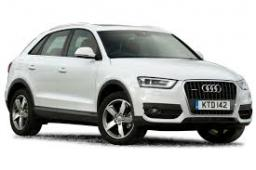Did you find an error or inaccuracy? Feel free to write us. Thank you!Tips to related online calculators
Check out our ratio calculator.
Do you have a system of equations and looking for calculator system of linear equations?

## Related math problems and questions:

• Ratio6 numbers are in the ratio 1:5:1:5:5:5. Their sum is 242. What are the numbers?
• Ratio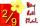Write the ratio with other numbers so that the value is the same: 2: 9
• Two squaresTwo squares whose sides are in the ratio 5:2 have a sum of its perimeters 73 cm. Calculate the sum of the area of these two squares.
• Change the numbers in the ratio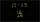Change the numbers 29, 38, and 43 in a 3: 4 ratio.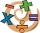Add six to twelve, then divide the sum by four. Divide the quotient by negative nine and then multiply by twenty-four. What is the result?
• Three numbers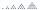What are three numbers that have the property: the sum of the first and second numbers' reciprocals is 12/7, the first and third 11/24, and the second and the third 3/8.
• Photo egative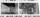Negative dimensions are 36mm and 28mm. What will be the photo size in the 21:4 ratio?
• Ornamental shrubsChildren committed to planting 240 ornamental shrubs. Their commitment, however, exceeded by 48 shrubs. Write the ratio of actually planted shrubs and commitment by lowest possible integers a/b.
• Numbers at ratio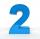The two numbers are in a ratio 3:2. If we each increase by 5 would be at a ratio of 4:3. What is the sum of original numbers?
• NumbersDetermine the number of all positive integers less than 4183444 if each is divisible by 29, 7, 17. What is its sum?
• Jan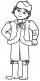Jan is 13 years and Peter 21 years old. For how many years will their ages in the ratio of 7:9?
• Scale of the mapDetermine the map's scale, which is the actual distance of 120 km l represented by a segment long 6 cm.
• PamelaLara Pamela spends her salary of 3000 for food, clothing, recreation and savings, which are in the ratio of 48:20:15:37, respectively. How much does he spend for savings?
• Money split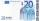Anton and Ferko got 2,500 euros together. The amount was split in a 1:4 ratio. How much did each get?
• Land area 2A land area was divided among the three heirs in the ratio 5:2:4. If the largest share was 20 hectares of land, what is the total area of land? Please show your solution and what kind of proportion is this please
• Unknown numberSamuel wrote unknown number. Then he had add 200000 to the number and the result multiply by three. When it calculated he was surprised, because the result would have received anyway, if write digit to the end of original number. Find unknown number.
• RatiosReduce the numbers: 50 in a 1:2 ratio 111 at a ratio of 2:3 70 at 10:50 560 at a ratio of 3:8